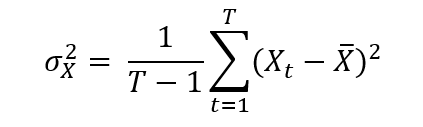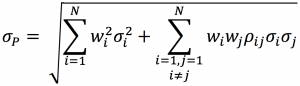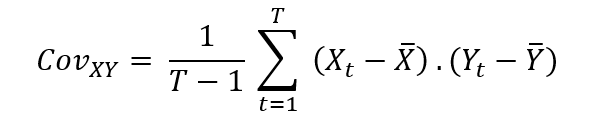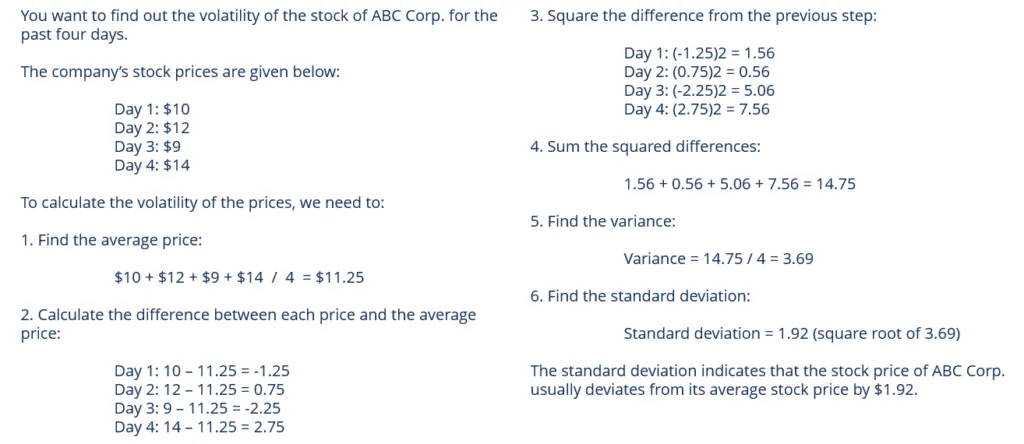# LWL | The Effect Volatility Has on Value at Risk

### Keywords: Volatility, Risk Forecast, Historical Volatility, Implied Volatility

Introduction

Investors utilize diverse methods to calculate what is known as Value at Risk (henceforth, VaR). The VaR, as Will Kenton (2022) explains, “is a statistic that calibrates the scope of potential financial losses over a specific time frame which enables risk managers to measure and control the level of risk exposure over a firm, position, or security.” Volatility is an essential factor that alters the outcome of the VaR as it is, in Hayes’s (2021) words, the sum of risk related to the magnitude of changes in a security’s value. In his article, Hayes (2021) explains that greater volatility means that the cost of an expense can potentially be spread over a wider range of values. Lower volatility means that the cost of an acquisition doesn't fluctuate widely and tends to be more constant. These are the two most common types of volatility according to Shubham Agarwal (2021) - historical volatility and implied volatility. Implied and historical volatility are the most utilized. The International Journal of Forecasting mentions exams on the S&P 500, Dow Jones Industrial Average and Nasdaq 100 indices over the course of more than twenty years with results comparing how historical volatility models outperform implied volatility models.

Types of volatility

Historical volatility is based on historical costs and represents the degree of fluctuation in an asset's returns. However, while variance generally captures the spread of returns around an asset's mean, volatility is a measure of this variance increased over a period of time.

Implied volatility (IV) calculates probability, demonstrates how volatile the market may become in the future, and is mainly used in options trading to determine the plausibility of a stock gaining a set price by a definite amount of time. However, this data is theoretical and as useful as the information may be. IV doesn’t provide the market direction and is not guaranteed to be correct.

All information gathered on the types of volatility are provided by Hayes (2021).

How to calculate Value at Risk.

According to Harper (2022), there are three main methods of assessing the Value at Risk.

Historical method

The first is called the historical method, which is the simplest method for computing VaR. Walia (2022) explains the historical method is based on the procedure of the pattern of historical returns being representative of the pattern of future returns.

This technique begins by collecting data on actions in market variables over a period of time. These variables include equity prices, interest rates, commodity prices, and more. Taking into account the daily price changes of the specific stock market index over the past 512 trading days, 512 cases have been collected that will perform as a guide of what will occur in the future movements of the index.

The percentage change in price for the index is calculated for each day. This defines the probability of distribution for daily gains or losses also known as the daily rate of returns. This is expressed as the formula:

Rt = Pt - Pt-1 / Pt-1

In which Rt represents the return over the period of time (t -, t) and Pt the price at time (t).

The historical returns are then sorted in order of worst-to-best observed returns over a period of time. The VaR can now be explained for the index in a time period of one day based on probability. The information provided on the historical method of calculating VaR was gathered from an article written by Walia (2022).

Variance-covariance method

The second method is the variance-covariance method. It utilizes an asset’s variances and covariances, and as it depends on the parameters of changes in price, it is referred to as a Parametric Method. The method assumes that an asset’s returns are commonly allocated surrounding the mean of the probability distribution. However, it also conjectures that the volatility of asset returns and the correlation between the asset returns are continuous over periods of time.

Calculating the VaR for a single asset is done by estimating the standard deviation (σ) under a specific time frame with the selected level of probability. This can be conveyed as:

VaR = a ⋅ σ

“Where the parameter ɑ links the quantile of the normal distribution and the standard deviation: ɑ = 2.33 for p = 99% and ɑ = 1.645 for p = 90%” (Walia, 2021).

Evaluating the VaR for a portfolio (P) of (N) assets begins by assessing the variance-covariance matrix. Asset X variance of returns is demonstrated as:The covariance between the returns of two assets X and Y measures how they vary with each other. Xt and Yt are returns for their respective assets and are shown as:The correlation coefficients are calculated as:

PXY = COVXY / σXσY

The volatility of portfolio (P) is computed with the usage of this formula:“Where wi corresponds to portfolio weights of asset i” (WALIA, 2021).

The following formula is utilized to calculate the Value at Risk of the portfolio:

VaR = a ⋅ σP

The quotes, images, and information provided on the variance-covariance method of calculating VaR was gathered from an article written by Walia (2021).

Monte Carlo Simulation

The third most common method used to calculate VaR is the monte carlo simulation. It is an extensive division of computational algorithms that depend on random repetitive instances in order to obtain numerical results. Its purpose is to create a prototype that displays the variety of possible results of an uncertain occurrence. This model aids in understanding the major risk and uncertainty impact.

This method covers a wide range of plausible layouts. The approach consists of running a large number of simulations of a random process repeatedly for a variable of interest. This may include asset returns as the variables are drawn from the analytical function, its parameters, and previously specified probability placements that are presumed to be known. These simulations permanently attempt to restore the share of return of an offer, from which Value at Risk can be calculated.

The monte carlo simulations begin by selecting a stochastic model for the conduct of the random variable of the specific index. This method is pertinent when the stochastic model and or the asset are of a complex nature, which leads to problems in assessing the VaR.

Walia (2022, para 5) explains, “For example, if we assume that returns follow a GARCH process, the (unconditional) VaR has to be computed with the Monte Carlo simulation methods. Similarly, if we consider complex financial products like options, the VaR has to be computed with the Monte Carlo simulation methods”.

The information provided on the monte carlo simulation method of calculating VaR was gathered from an article written by Walia (2022).

How to calculate Volatility

Hayes (2021) explains how volatility is calculated in the most efficient and precise way utilizing variance and standard deviation which is the square root of the variance. These measures vary depending on which type of volatility is being computed.

Historical volatility

Historical volatility evaluates the distribution of returns of a firm, security, or index over a specific time period. This process begins by collecting the historical prices for the specified asset, which is then followed by calculating the mean, also known as the expected price, of the collected historical prices. Afterwards, the difference between each price and the average price is computed and the difference is squared. The sums of the squared differences are determined and divided by the total number of prices in order to discover the variance. Finally, the square root of the variance is assessed and the standard deviation has been calculated.Figure 1: Method for calculating price volatility
Source: Corporate Financial Institute (CFI).

Implied volatility

Implied volatility is calculated using the following formula:

C = SN (d1) – N (d2) Ke -rt

Where C is the Option Premium, S is the price of the stock, K is the Strike Price, r is the risk-free rate, t is the time to maturity, and e is the exponential term. This information was gathered and provided by Harsh Katara.

Implied volatility vs. historical volatility

Accurate Value at Risk forecasts are provided by the evaluation and comparison of implied volatility and historical volatility models. Daily data was collected over the past twenty years through experimental tests and observations made on the S&P 500. The information was gathered by Dennis Bams (2017) focusing on the Dow Jones Industrial Average and Nasdaq 100 and over time indicated that Value at Risk based on implied volatility tends to be exceeded by a Value at Risk based on a modest GJR-GARCH. These discoveries have become a sturdy pillar to the dynamic quantile test for gauging the execution of the Value at Risk also referred to as the likelihood ratio.

Volatility risk contains a negative price for which both nonparametric and parametric accommodations are manufactured. This negative price is tied with implied volatility and is the cause of the inferior results shown compared to the historical volatility GJR-GARCH models.

Volatility forecasting prefers implied volatility models over historical volatility models, which contrast with the results of the S&P 500 index on the Dow Jones Industrial Average and Nasdaq 100 evaluation. There are two main purposes of the nonlinear volatility that include (i) forecasting the volatility and (ii) forecasting a quantile of the return administration. Of these two main objectives, implied volatility models have only proven to be efficient when forecasting volatility which is ever changing and never consistent.

The information provided on the similarities and differences of implied and historical volatility are written by Dennis Bams, Gildas Blanchard, and Thorsten Lehnert (2017).

Conclusion

There exist different types of volatility and each contain their own diverse measures of calculation and purpose. However, they all prove to have a significant effect on the results of the value at risk calculations. Historical and implied volatility are the most widely used and recently proved to outperform implied volatility models. The three main methods of calculating the value at risk were major factors in proving the effect of volatility. These are the calculations and measures taken by investors who desire to dive into the extensive concept that is known as value at risk.

Bibliography

Bams, D., Blanchard, G., & Lehnert, T. (2017). Volatility measures and value-at-risk. International Journal of Forecasting. July 24. Retrieved March 27, 2022, from https://www.sciencedirect.com/science/article/abs/pii/S0169207017300511

Ganti, A. (2022). How implied volatility (IV) helps you to buy low and sell high. Investopedia. February 8. Retrieved April 16, 2022, from https://www.investopedia.com/terms/i/iv.asp

Harper, D. R. (2022). An introduction to value at risk (VAR). Investopedia. March 24. Retrieved March 27, 2022, from https://www.investopedia.com/articles/04/092904.asp

Hayes, A. (2022). Volatility. Investopedia. February 8. Retrieved March 27, 2022, from https://www.investopedia.com/terms/v/volatility.asp

Katara, H. (2021). Implied volatility formula. WallStreetMojo. September 20. Retrieved April 20, 2022, from https://www.wallstreetmojo.com/implied-volatility-formula/

Kenton, W. (2022). Value at risk (VAR) Explained. Investopedia. March 10. Retrieved March 27, 2022, from https://www.investopedia.com/terms/v/var.asp